xBoundary value problemOverview

In mathematics
Mathematics
Mathematics is the study of quantity, space, structure, and change. Mathematicians seek out patterns and formulate new conjectures. Mathematicians resolve the truth or falsity of conjectures by mathematical proofs, which are arguments sufficient to convince other mathematicians of their validity...

, in the field of differential equation
Differential equation
A differential equation is a mathematical equation for an unknown function of one or several variables that relates the values of the function itself and its derivatives of various orders...

s, a boundary value problem is a differential equation
Differential equation
A differential equation is a mathematical equation for an unknown function of one or several variables that relates the values of the function itself and its derivatives of various orders...

together with a set of additional restraints, called the boundary conditions. A solution to a boundary value problem is a solution to the differential equation which also satisfies the boundary conditions.

Boundary value problems arise in several branches of physics as any physical differential equation will have them.Unanswered QuestionsEncyclopedia
In mathematics
Mathematics
Mathematics is the study of quantity, space, structure, and change. Mathematicians seek out patterns and formulate new conjectures. Mathematicians resolve the truth or falsity of conjectures by mathematical proofs, which are arguments sufficient to convince other mathematicians of their validity...

, in the field of differential equation
Differential equation
A differential equation is a mathematical equation for an unknown function of one or several variables that relates the values of the function itself and its derivatives of various orders...

s, a boundary value problem is a differential equation
Differential equation
A differential equation is a mathematical equation for an unknown function of one or several variables that relates the values of the function itself and its derivatives of various orders...

together with a set of additional restraints, called the boundary conditions. A solution to a boundary value problem is a solution to the differential equation which also satisfies the boundary conditions.

Boundary value problems arise in several branches of physics as any physical differential equation will have them. Problems involving the wave equation
Wave equation
The wave equation is an important second-order linear partial differential equation for the description of waves – as they occur in physics – such as sound waves, light waves and water waves. It arises in fields like acoustics, electromagnetics, and fluid dynamics...

, such as the determination of normal mode
Normal mode
A normal mode of an oscillating system is a pattern of motion in which all parts of the system move sinusoidally with the same frequency and with a fixed phase relation. The frequencies of the normal modes of a system are known as its natural frequencies or resonant frequencies...

s, are often stated as boundary value problems. A large class of important boundary value problems are the Sturm–Liouville problems. The analysis of these problems involves the eigenfunction
Eigenfunction
In mathematics, an eigenfunction of a linear operator, A, defined on some function space is any non-zero function f in that space that returns from the operator exactly as is, except for a multiplicative scaling factor. More precisely, one has...

s of a differential operator
Differential operator
In mathematics, a differential operator is an operator defined as a function of the differentiation operator. It is helpful, as a matter of notation first, to consider differentiation as an abstract operation, accepting a function and returning another .This article considers only linear operators,...

.

To be useful in applications, a boundary value problem should be well posed
Well-posed problem
The mathematical term well-posed problem stems from a definition given by Jacques Hadamard. He believed that mathematical models of physical phenomena should have the properties that# A solution exists# The solution is unique...

. This means that given the input to the problem there exists a unique solution, which depends continuously on the input. Much theoretical work in the field of partial differential equation
Partial differential equation
In mathematics, partial differential equations are a type of differential equation, i.e., a relation involving an unknown function of several independent variables and their partial derivatives with respect to those variables...

s is devoted to proving that boundary value problems arising from scientific and engineering applications are in fact well-posed.

Among the earliest boundary value problems to be studied is the Dirichlet problem
Dirichlet problem
In mathematics, a Dirichlet problem is the problem of finding a function which solves a specified partial differential equation in the interior of a given region that takes prescribed values on the boundary of the region....

, of finding the harmonic function
Harmonic function
In mathematics, mathematical physics and the theory of stochastic processes, a harmonic function is a twice continuously differentiable function f : U → R which satisfies Laplace's equation, i.e....

s (solutions to Laplace's equation
Laplace's equation
In mathematics, Laplace's equation is a second-order partial differential equation named after Pierre-Simon Laplace who first studied its properties. This is often written as:where ∆ = ∇² is the Laplace operator and \varphi is a scalar function...

); the solution was given by the Dirichlet's principle.

## Initial value problem

A more mathematical way to picture the difference between an initial value problem and a boundary value problem is that an initial value problem has all of the conditions specified at the same value of the independent variable in the equation (and that value is at the lower boundary of the domain, thus the term "initial" value). On the other hand, a boundary value problem has conditions specified at the extremes of the independent variable. For example, if the independent variable is time over the domain [0,1], an initial value problem would specify a value of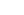and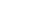at time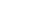, while a boundary value problem would specify values forat bothand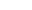.

If the problem is dependent on both space and time, then instead of specifying the value of the problem at a given point for all time the data could be given at a given time for all space. For example, the temperature of an iron bar with one end kept at absolute zero
Absolute zero
Absolute zero is the theoretical temperature at which entropy reaches its minimum value. The laws of thermodynamics state that absolute zero cannot be reached using only thermodynamic means....

and the other end at the freezing point of water would be a boundary value problem.

Concretely, an example of a boundary value (in one spatial dimension) is the problem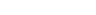to be solved for the unknown function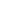with the boundary conditions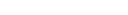Without the boundary conditions, the general solution to this equation is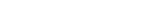From the boundary condition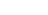one obtains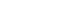which implies that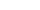From the boundary condition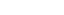one finds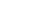and so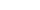One sees that imposing boundary conditions allowed one to determine a unique solution, which in this case is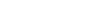## Types of boundary value problems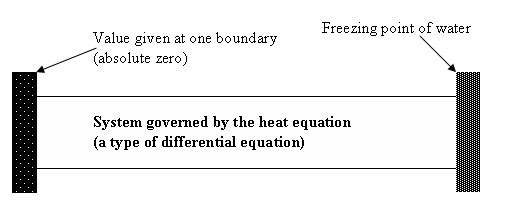If the boundary gives a value to the normal derivative of the problem then it is a Neumann boundary condition
Neumann boundary condition
In mathematics, the Neumann boundary condition is a type of boundary condition, named after Carl Neumann.When imposed on an ordinary or a partial differential equation, it specifies the values that the derivative of a solution is to take on the boundary of the domain.* For an ordinary...

. For example, if there is a heater at one end of an iron rod, then energy would be added at a constant rate but the actual temperature would not be known.

If the boundary gives a value to the problem then it is a Dirichlet boundary condition
Dirichlet boundary condition
In mathematics, the Dirichlet boundary condition is a type of boundary condition, named after Johann Peter Gustav Lejeune Dirichlet who studied under Cauchy and succeeded Gauss at University of Göttingen. When imposed on an ordinary or a partial differential equation, it specifies the values a...

. For example, if one end of an iron rod is held at absolute zero, then the value of the problem would be known at that point in space.

If the boundary has the form of a curve or surface that gives a value to the normal derivative and the problem itself then it is a Cauchy boundary condition
Cauchy boundary condition
In mathematics, a Cauchy boundary condition imposed on an ordinary differential equation or a partial differential equation specifies both the values a solution of a differential equation is to take on the boundary of the domain and the normal derivative at the boundary. It corresponds to imposing...

.

Aside from the boundary condition, boundary value problems are also classified according to the type of differential operator involved. For an elliptic operator
Elliptic operator
In the theory of partial differential equations, elliptic operators are differential operators that generalize the Laplace operator. They are defined by the condition that the coefficients of the highest-order derivatives be positive, which implies the key property that the principal symbol is...

, one discusses elliptic boundary value problem
Elliptic boundary value problem
In mathematics, an elliptic boundary value problem is a special kind of boundary value problem which can be thought of as the stable state of an evolution problem...

s. For an hyperbolic operator, one discusses hyperbolic boundary value problems. These categories are further subdivided into linear and various nonlinear types.

## See also

Related mathematics:
• initial value problem
Initial value problem
In mathematics, in the field of differential equations, an initial value problem is an ordinary differential equation together with a specified value, called the initial condition, of the unknown function at a given point in the domain of the solution...

• differential equation
Differential equation
A differential equation is a mathematical equation for an unknown function of one or several variables that relates the values of the function itself and its derivatives of various orders...

s
• Green's function
Green's function
In mathematics, a Green's function is a type of function used to solve inhomogeneous differential equations subject to specific initial conditions or boundary conditions...

s
• Stochastic processes and boundary value problems
Stochastic processes and boundary value problems
In mathematics, some boundary value problems can be solved using the methods of stochastic analysis. Perhaps the most celebrated example is Shizuo Kakutani's 1944 solution of the Dirichlet problem for the Laplace operator using Brownian motion...

• Sturm–Liouville theory
• Dirichlet boundary condition
Dirichlet boundary condition
In mathematics, the Dirichlet boundary condition is a type of boundary condition, named after Johann Peter Gustav Lejeune Dirichlet who studied under Cauchy and succeeded Gauss at University of Göttingen. When imposed on an ordinary or a partial differential equation, it specifies the values a...

• Neumann boundary condition
Neumann boundary condition
In mathematics, the Neumann boundary condition is a type of boundary condition, named after Carl Neumann.When imposed on an ordinary or a partial differential equation, it specifies the values that the derivative of a solution is to take on the boundary of the domain.* For an ordinary...

• Robin boundary condition
Robin boundary condition
In mathematics, the Robin boundary condition is a type of boundary condition, named after Victor Gustave Robin . When imposed on an ordinary or a partial differential equation, it is a specification of a linear combination of the values of a function and the values of its derivative on the...

• Sommerfeld radiation condition
• Cauchy boundary condition
Cauchy boundary condition
In mathematics, a Cauchy boundary condition imposed on an ordinary differential equation or a partial differential equation specifies both the values a solution of a differential equation is to take on the boundary of the domain and the normal derivative at the boundary. It corresponds to imposing...

• Mixed boundary condition
Mixed boundary condition
In mathematics, a mixed boundary condition for a partial differential equation indicates that different boundary conditions are used on different parts of the boundary of the domain of the equation....

Physical applications:
• wave
Wave
In physics, a wave is a disturbance that travels through space and time, accompanied by the transfer of energy.Waves travel and the wave motion transfers energy from one point to another, often with no permanent displacement of the particles of the medium—that is, with little or no associated mass...

s
• normal mode
Normal mode
A normal mode of an oscillating system is a pattern of motion in which all parts of the system move sinusoidally with the same frequency and with a fixed phase relation. The frequencies of the normal modes of a system are known as its natural frequencies or resonant frequencies...

s
• electrostatics
Electrostatics
Electrostatics is the branch of physics that deals with the phenomena and properties of stationary or slow-moving electric charges....

• Laplace's equation
Laplace's equation
In mathematics, Laplace's equation is a second-order partial differential equation named after Pierre-Simon Laplace who first studied its properties. This is often written as:where ∆ = ∇² is the Laplace operator and \varphi is a scalar function...

• potential theory
Potential theory
In mathematics and mathematical physics, potential theory may be defined as the study of harmonic functions.- Definition and comments :The term "potential theory" was coined in 19th-century physics, when it was realized that the fundamental forces of nature could be modeled using potentials which...

• Computation of radiowave attenuation in the atmosphere
Computation of radiowave attenuation in the atmosphere
One of the causes of attenuation of radio propagation is the absorption by the atmosphere. There are many well known facts on the phenomenon and qualitative treatments in textbooks. A document published by the International Telecommunication Union...

• Black holes

Numerical algorithms:
• shooting method
Shooting method
In numerical analysis, the shooting method is a method for solving a boundary value problem by reducing it to the solution of an initial value problem...

• direct multiple shooting method
Direct multiple shooting method
In the area of mathematics known as numerical ordinary differential equations, the direct multiple shooting method is a numerical method for the solution of boundary value problems...

## External links

The source of this article is wikipedia, the free encyclopedia.  The text of this article is licensed under the GFDL.